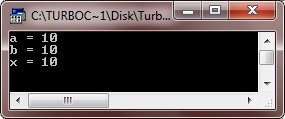# C++ Assignment Operator and Statement

## Assignment Operator

Basically, assignment operator is used to assign the value of one variable to another variable. Or assign value to a variable. Here is the general form to assign variable's value or value to a variable

```a = b;
x = 10;```

In the above statements, the value of b is assigned to a, and the value 10 is assigned to x. Here is an example

```/* C++ Assignment Operator */

#include<iostream.h>
#include<conio.h>
void main()
{
clrscr();

int a, b=10, x;
a = b;
x = 10;

cout<<"a = "<<a<<endl;
cout<<"b = "<<b<<endl;
cout<<"x = "<<x<<endl;

getch();
}```

Here is the sample output of the above C++ program:## Assignment Statement

As you know, an expression is composed of one or more operations. An expression terminated ; (semicolon) becomes a statement. Statements from the smallest executable unit within a C++ program. Statements are terminated with a semicolon.

An assignment statement assigns value to a variable. The value assigned may be constant, variable or an expression. The general form of an assignment statement is as follows:

`a = cve ;`

where a is a variable to whom the value is being assigned and cve can either be a constant or variable or an expression. Following are some examples of assignment statements :

```x = 16;
y = 3.6;
z = x + y;
z = z × x;
a = b + c - d;```

The symbol "=" is called as the assignment operator. One usual feature of C++ is that you can use the assignment operator serially.

Tip - Assignments can be chained together.

The assignment operator = returns the value of the assignment as well as actually assigning the value to the left hand operand. Because of that, assignments can be chained together. This can be useful when assigning the same value to a number of items. For example,

`x = y = z = 13`

This statement assigns the value 13 to x, y, and z. All the variables in this multiple assignment statement must be declared before. Such statement works from right to left. First, 13 is assigned to z, then the value of z, which is now 13, assigned to y, then y's value of 13 is assigned to x.

Attention - The TurboC++ version 3.0 allows to chain maximum 70 assignments. All the variables participating in the multiple assignment statement must be predeclared.

### More Examples

Here are some C++ programs listed, that you may like:

Tools
Calculator